# Weighing the Earth in 1798: The Cavendish Experiment

## Victoria Chang October 31, 2007

### (Submitted as coursework for Physics 210, Stanford University, Fall 2007)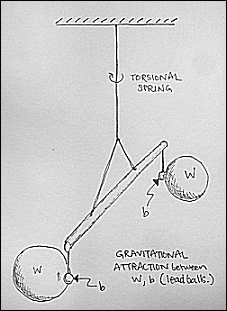Fig. 1: Schematic of apparatus. With the large balls W held in fixed position, the small balls b feel two opposing forces, one due to gravitational attraction to the large balls, and one due to the restoring torque from the suspension wire, which acts as a torsional spring.

Henry Cavendish's experiments determining the density of the Earth were published in the Philosophical Transactions of the Royal Society in 1798. His method, following a procedure obtained from his friend John Michell, consisted of using a torsional spring to find the gravitational force between lead spheres smaller than 1 foot in diameter. In doing so he not only found the mass of the Earth, which then yielded masses for other celestial objects such as the Sun and Moon, but also verified the universal nature of Newton's Law of Gravitation. At that time Newton's law had proven to be of ample use in predicting the motion of the planets (Falconer), but as Cavendish notes in the opening of his paper, an important merit of Michell's procedure was its ability to "[render] sensible the attraction of small quantities of matter" (Cavendish), thereby bringing the inverse square law down from celestial orbits and into the laboratory. Famously, we can use Cavendish's results to calculate the gravitational constant G; the Cavendish experiment is, in fact, commonly described as the first determination of this constant, though the man himself entirely avoids it. As we shall see, virtually all calculations are done via ratios so that proportionality constants are, in general, dropped out; this practice was common as standard units had not been set at that time.

## Concepts Behind the Procedure

Here I will present a very simplified analysis of the experiment, which will provide the reader with a basic idea of the concepts at work. In the following sections I will describe some of the corrections to this simplified view that allowed for such a precise measurement. A torsional spring is analogous to the familiar linear mass on a spring, in which Hooke's law is rewritten as

τ = – k θ

so that the restoring torque τ exerted by the spring is proportional (by spring constant k) to the angular displacement θ by which it is twisted. The analogue of mass in a torsional system is the moment of inertia I of the system, and so the period of oscillation, assuming that there are no dissipative forces and that the mass of the spring itself is negligible, is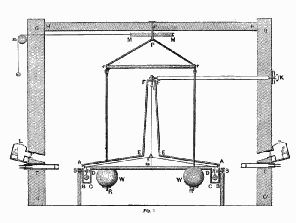Fig. 2: Cross-section of actual apparatus. This is a figure from Cavendish's paper depicting a cross-section of the building housing his apparatus. The torsional system is enclosed in a wooden case to shield it from wind, and its angular displacement is determined via telescopes (lighting provided by lamps). The large balls are hung from a pulley system via copper rods. The angular displacement of the supporting rod is measured via ivory slips attached to the case, etched with 1/20" divisions, and ivory verniers attached to each end of the rod, subdividing the divisions into 5 parts. The building remains closed during each experiment. Taken from Wikimedia.org.
T = 2π (I / k)1/2

Thus if we allow the system to oscillate at its resonant frequency 1/T, we can find k. Then, if we apply an unknown external torque, we may find it by allowing the system to come to equilibrium and then measuring the equilibrium angular displacement θeq, since the external torque is simply equal to k θeq.

In the experiment at hand, the moment of inertia of the system is approximately that of two point masses, each with mass equal to mb (the mass of each small ball b), located at the ends of the supporting rod (whose mass is ignored for now). If the large balls W are absent, there is no external torque on the system and the angular displacement is zero. Once the large balls are brought close to the small balls in the orientation shown in Fig. 1, there is an (unknown) external torque due to the gravitational attraction between the neighboring large/small pairs. This torque will be very small! Apparently the sensitivity of this experiment depends on the fact that the spring constant k is also very small, so that the resulting angular displacement is large enough to detect. A longer supporting rod will also aid detection since the spatial displacement of its ends will consequently be larger.

Now, assuming that we place the large balls in such a way that the line between the centers of the neighboring large/small pairs is perpendicular to the supporting rod, the torque equation at equilibrium is

GmWmb/rWb2 = k θeq/L

where L is the length of the supporting rod and rWb is the distance between the centers of each large/small pair. In theory we know all the values in this equation from the experiment, except G.

## Details of the Apparatus

Let's make a note of some of the assumptions made in the simplified view above. We assumed no dissipative forces in the spring (so that the period of oscillation actually gives the resonant frequency of the system). This doesn't turn out to be such a bad approximation since the oscillations are so small and slow (due to the tiny spring constant and tiny external torque). We assumed that the only source of moment of inertia was due to the small balls, which we approximated as point masses. We'll have to correct for that, because the supporting rod has a non-negligible mass, and the small balls are not point masses. (The mass of the suspension wire used in at the majority of the experiments is not given in the paper, but we can assume that it is negligible.)

 Mass of large balls mW 2439000 grains 158.04 kg Mass of small balls mb 11262 grains 0.73 kg Mass of supporting rod - inertial (equivalent small ball mass) mI,rod 398 grains .03 kg Mass of supporting rod - gravitational (equivalent small ball mass) mG,rod 157 grains .01 kg Mass of copper rods (equivalent small ball mass) mcopper 18800 grains 1.22 kg Distance between large balls L 73.30 in 1.860 m Distance between small balls (length of supporting rod) L 73.30 in 1.860 m Distance between ivory veniers at ends of supporting rod Li 76.60 in 1.950 m Radius of small balls rb 1.00 in .025 m Closest distance between large/small centers d 8.85 in .225 m
Table 1: Experimental parameters.

Now for a few details that are particular to the experimental set-up. Table 1 gives an account of all masses and distances that entered the calculations and (non-negligible) corrections.

All of these values were found in Cavendish's paper. He describes how he determined the effective masses in great detail, and I will not repeat those calculations - it suffices to say that many integrals were done. It is interesting that the effective mass of the supporting rod differs depending on whether we are considering its inertial mass or its gravitational mass. I haven't thought about that very much, but I should.

## Method of Analysis

The ivory slips and verniers, which allowed for a measurement of the vernier's displacement to within 1/100" (1/20" per division on the slip divided by 5 additional divisions on the vernier), were placed so that an angular displacement of the system produced a division displacement of the same sign on both ends of the rod. The pulley system suspending the large balls could be rotated, so that bringing the large balls close to the small ones from one direction would produce a positive division displacement (+), whereas bringing them close from the opposite direction would produce a negative displacement(-). See Fig. 3.

To determine the angular displacement of the torsional system, Cavendish found that the most effective method was to start with the large balls in either extreme position, (+) or (-), allow the vibrations to decay until the system was more-or-less at rest, and then move the large balls to the opposite extreme position. In this manner the torsional system would begin at its equilibrium angular displacement ± θeq with a potential energy proportional to θeq2; upon arrival of the large balls at the opposite extreme position, the system would begin to oscillate, having been given an extra initial amount of stored potential energy. The final equilibrium position, called the "point of rest", was determined by first taking the average of the first and third extremities of the vibration, and then taking the average of that value and the second extremity. The time of vibration was determined by choosing a fixed point and measuring the time between successive returns to that point, divided by the number of vibrations during that interval. (It seems to me that what was actually being measured was a half-period.)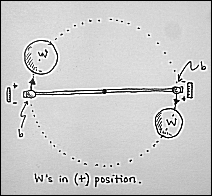Fig. 3: Positioning of large balls. We look at the apparatus from above. Here the large balls are depicted in the (+) position, so that the gravitational force between each large/small pair (W and b) draws each small ball in the positive direction along the ivory slip, producing a positive division displacement (i.e. a CW angular displacement). Note that the gravitational force on the small balls is not exactly perpendicular to the rod, so the torque imparted on the torsional system is a little less than simply FgravL/2.

Each trial thus provides a value for the time of vibration (in seconds) N of the suspension wire (which corresponds to the period of oscillation) and the number of divisions B by which the ivory verniers at the supporting rod's ends has been displaced (which corresponds to the angular displacement of the torsional system). Cavendish then used each pair of results not to calculate the gravitational constant G (as described in part I of this report) but rather to find the density of the earth. How did he go about doing this?

Rather than determining the spring constant k directly, he modelled the oscillations of the torsional system as the swings of a pendulum with length equal to L/2, half the length of the supporting rod. Then the period of oscillation can be expressed either as 2π (L/2g)1/2 or 2π (I/k)1/2, where I = m(L/2)2, m being the effective mass at the end of the rod. Setting these equal and multiplying through by θ, we find:

Fθ/(mg) = θL/2

where Fθ = kθ is the force required for an angular displacement of θ. Cavendish found the period of oscillation by comparing this pendulum with a pendulum whose period was known to be 1 second, whose length was 39.14 in; then the period N of our torsional system is simply

N = [(L/2)/39.14 in]1/2 seconds

so that

Fθ/(mg) = (θL/2)((L/2)/39.14)/N2

where we've just multiplied the right side by 1 = ((L/2)/39.14)/N2. We want θ in terms of the division displacement B, remembering that each division corresponds to 1/20". But we can approximate &theta = sin-1((B/20)/(L/2)) ≈ (B/20)/(L/2) at small angles. Plugging in for L (in inches so all units cancel out), we finally get

 Fθ/(mg) = (B/766)(36.65/39.14)/N2 ⇒ Fθ/(mg) = B/818N2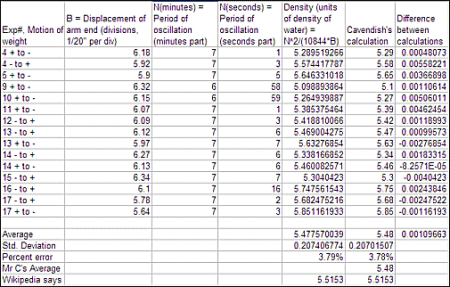Table 2: Final Results. The following table shows the results for B, N, and ρE for all experiments. The actual value used for B is that shown divided by 2, since the displacement in these experiments is from one extreme to the other and we want the displacement from the point of rest.

The final step gets us the density of the earth ρE which is given in units of water density. The large balls each have mass equal to that of 10.66 spheres of water with diameter 1 foot each, which one can check easily. Cavendish knew the mean diameter of the Earth to be 41800000 feet as the method for calculating this value via trigonometry had been known since Eratosthenes. Then we take the ratio of the gravitational forces on each small ball due to (1) each large ball, which is 8.85 inches away, vs. (2) the Earth at its surface, will be

FW / FEarth = (ρE/10.66 gm cm-3) (dE/1 foot) (6 in/8.85 in)2

(the last factor comes from the fact that the large balls are actually 8.85 inches away from the small balls (center-to-center), rather than 6 inches as they would be if they were spheres of water with diameter 1 foot). We must include one extra small factor which accounts for the fact that the large balls are not directly perpendicular, center-to-center, to the supporting rod, which is 0.9779. We set Fθ equal to FW and use the fact that FEarth = mg to find, finally, that

ρE = N2/10844B

I have left out just one more correction that was used in reaching this final result, the gravitational attraction between the opposite large/small pairs, which opposes the dominant force and reduces the actual attraction by a factor of .9983. The calculation of this factor is described in detail by Cavendish.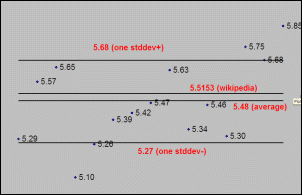Fig. 4: Scatter plot of results.

## Cavendish's Final Results

Table 2 and Fig. 4 present the results of this year-long endeavor. The first is a tabulation of results from selected experiments. The second is a graph of these results along with the calculated average alongside today's accepted value for the density of the Earth.

Today's standard value for the density of the earth (5.5153 g/cm3) is well within one standard deviation of Cavendish's average of 5.48 g/cm3.

© Victoria Chang. The author grants permission to copy, distribute and display this work in unaltered form, with attributation to the author, for noncommercial purposes only. All other rights, including commercial rights, are reserved to the author.

## References

 H. Cavendish, Phil. Trans. Roy. Soc. 88, 469 (1798).

 I. Falconer, Meas. Sci. Tech. 10, 470 (1999).